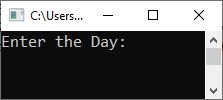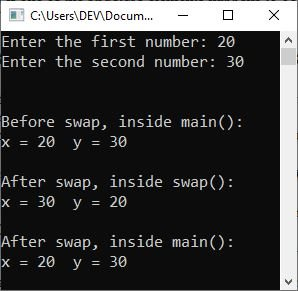# C++ Passing Structure to Function

So far, all structures used in the preceding examples have been global and hence available to all the functions within the program. But if you have a structure local to a function and you need to pass its values to another function, then it can be achieved in two ways:

Using either the call-by-value method or the call-by-reference method to pass variables is an option for accomplishing either of these two ways of getting the job done. Let's go into further detail about these, shall we?

## Passing structure elements to a function in C++

When an element of a structure is passed to a function, you are actually passing the values of that element to the function. Therefore, it is just like passing a simple variable (unless, of course, that element is complex, such as an array of characters). For example, consider the following structure:

```struct date {
int day;
int month;
int year;
}dob;```

Individual elements of this structure can be passed as follows:

`myFunction(dob.day, dob.month, dob.year);`

The above function-call invokes a function named "myFunction()" by passing values for individual structure elements using the structure variable "dob." As an example:

```#include<iostream>
using namespace std;

struct date {
int day;
int month;
int year;
};

void printDob(int, int, int);       // function prototype

int main()
{
date dob;
cout<<"Enter the Day: ";
cin>>dob.day;
cout<<"Enter the Month: ";
cin>>dob.month;
cout<<"Enter the Year: ";
cin>>dob.year;

printDob(dob.day, dob.month, dob.year);       // function call

cout<<endl;
return 0;
}
void printDob(int a, int b, int c)       // function definition
{
cout<<"\nDate of Birth in DD-MM-YYYY Format = "<<a<<"-"<<b<<"-"<<c;
}```

The following snapshot shows the initial output produced by the above program:Now enter the following inputs: "12" as the day and press the ENTER key, "10" as the month and press the ENTER key again, and "2001" as the year and press the ENTER key to produce the following output:Let me describe the above program in brief.

• I defined a named structure "date" with three members of the "int" type, namely "day," "month," and "year" in the preceding example.
• I created a prototype of a function called "printDob()" that accepts three "int" parameters but returns null.
• I created the structure variable "dob" inside the "main()" method to access all three members of the structure "date."
• I use "cout" and "cin" to print the message to the output console and tell the user what to do, so the user enters the three values.
• In the structure member, I saved all three values: day, month, and year.
• Then I called the function "printDob()," passing it all three structure elements as parameters.
• All three parameters will be copied to the variables "a," "b," and "c" in the function definition section in this manner.
• The only thing I've done so far is print the values of all three.
• On the output console, variables are displayed.

The function can either receive the values by creating its own copy of them (call by value) or by creating references for the original variables (call by reference). If you don't want the function to change the values of the structure elements, pass them by value; if you want the function to change the original values, pass them by reference.

But remember, if one of the structure elements happens to be an array, it will automatically be passed by reference, as arrays cannot be passed by value.

### Passing structure elements to a function: call-by-value

Take a look at the following program, which shows how to use the call by value method to pass structure elements to a function:

```#include<iostream>
using namespace std;

struct myStructure {
int x;
int y;
};

void swap(int, int);

int main()
{
myStructure ms;
cout<<"Enter the first number: ";
cin>>ms.x;
cout<<"Enter the second number: ";
cin>>ms.y;

cout<<"\n\nBefore swap, inside main():\n";
cout<<"x = "<<ms.x<<"\ty = "<<ms.y;

swap(ms.x, ms.y);

cout<<"\n\nAfter swap, inside main():\n";
cout<<"x = "<<ms.x<<"\ty = "<<ms.y;

cout<<endl;
return 0;
}
void swap(int x, int y)
{
int temp = x;
x = y;
y = temp;

cout<<"\n\nAfter swap, inside swap():\n";
cout<<"x = "<<x<<"\ty = "<<y;
}```

The following snapshot shows the sample run of the above example program with user inputs of 20 and 30 as two numbers.In the above example, you can use other variable names instead of "x" and "y" in the function definition section.

### Passing structure elements to a function: call-by-reference

Now take a look at the following program, which shows how to use the call by reference method to pass structure elements to a function:

```#include<iostream>
using namespace std;

struct myStructure {
int x;
int y;
};

void swap(int &, int &);

int main()
{
myStructure ms;
cout<<"Enter the first number: ";
cin>>ms.x;
cout<<"Enter the second number: ";
cin>>ms.y;

cout<<"\n\nBefore swap, inside main():\n";
cout<<"x = "<<ms.x<<"\ty = "<<ms.y;

swap(ms.x, ms.y);

cout<<"\n\nAfter swap, inside main():\n";
cout<<"x = "<<ms.x<<"\ty = "<<ms.y;

cout<<endl;
return 0;
}
void swap(int &x, int &y)
{
int temp = x;
x = y;
y = temp;

cout<<"\n\nAfter swap, inside swap():\n";
cout<<"x = "<<x<<"\ty = "<<y;
}```

The sample run with the same users' inputs as the previous program should exactly be:I only used the "&" (address of) operator before the variables "x" and "y" in the function definition section and used the same operator while creating the function prototype. The rest of all the codes remain unchanged.

## In C++, passing the entire structure to a function

Passing entire structures makes the most sense when the structure is relatively compact. The entire structure can be passed to the functions both by value and by reference. Passing by value is useful when the original values are not to be changed, and passing by reference is useful when the original values are to be changed.

### Passing the entire structure to a function: call-by-value

When a structure is used as an argument to a function, the entire structure is passed using the standard call-by-value method. Of course, this means that any changes made to the contents of the structure inside the function to which it is passed do not affect the structure used as an argument.

The receiving parameter for the passed structure must match the type of the passed structure. As an example:

```#include<iostream>
using namespace std;

struct myStructure {
int x;
int y;
};

void swap(myStructure);

int main()
{
myStructure ms;
cout<<"Enter the first number: ";
cin>>ms.x;
cout<<"Enter the second number: ";
cin>>ms.y;

cout<<"\n\nBefore swap, inside main():\n";
cout<<"x = "<<ms.x<<"\ty = "<<ms.y;

swap(ms);

cout<<"\n\nAfter swap, inside main():\n";
cout<<"x = "<<ms.x<<"\ty = "<<ms.y;

cout<<endl;
return 0;
}
void swap(myStructure ms)
{
int temp = ms.x;
ms.x = ms.y;
ms.y = temp;

cout<<"\n\nAfter swap, inside swap():\n";
cout<<"x = "<<ms.x<<"\ty = "<<ms.y;
}```

The above program does the same job as the program given in the "call-by-value" section of "passing a structure element to a function" above.

### Passing the entire structure to a function: call-by-reference

Structures can be passed by reference just like other simple types. When a structure is passed by reference, the called function declares a reference for the passed structure and refers to the original structure elements through its reference. Thus, the called function works with the original values.

The following example program illustrates the passing of structures to functions with the call by reference method:

```#include<iostream>
using namespace std;

struct myStructure {
int x;
int y;
};

void swap(myStructure &);

int main()
{
myStructure ms;
cout<<"Enter the first number: ";
cin>>ms.x;
cout<<"Enter the second number: ";
cin>>ms.y;

cout<<"\n\nBefore swap, inside main():\n";
cout<<"x = "<<ms.x<<"\ty = "<<ms.y;

swap(ms);

cout<<"\n\nAfter swap, inside main():\n";
cout<<"x = "<<ms.x<<"\ty = "<<ms.y;

cout<<endl;
return 0;
}
void swap(myStructure &ms)
{
int temp = ms.x;
ms.x = ms.y;
ms.y = temp;

cout<<"\n\nAfter swap, inside swap():\n";
cout<<"x = "<<ms.x<<"\ty = "<<ms.y;
}```

The above program does the same job as the program given in the "call-by-reference" section of "passing a structure element to a function" above.

C++ Quiz

« Previous Tutorial Next Tutorial »